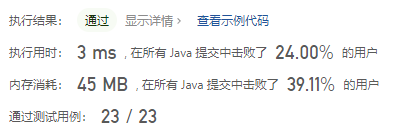#### 167. 两数之和 II - 输入有序数组

###### 来源: 每日一题 2023.07.08

``````输入：numbers = [2,7,11,15], target = 9

``````

``````输入：numbers = [2,3,4], target = 6

``````

``````输入：numbers = [-1,0], target = -1

``````

``````2 <= numbers.length <= 3 * 104
-1000 <= numbers[i] <= 1000
numbers 按 非递减顺序 排列
-1000 <= target <= 1000

``````
``````class Solution {
public int[] twoSum(int[] numbers, int target) {

}
}
``````

#### 分析与题解

• 二分法

这个题目和 力扣的第一题 `两数之和` 很类似, 但是这个题多了一个条件, 同时也多了一个要求.

• 条件: 该数组已按 非递减顺序排列 .

• 要求: 你所设计的解决方案必须只使用常量级的额外空间。

当题目中说数组是一个有序数组时,我想到的第一反应就是使用二分法解决这个问题, 我们只需要在一次遍历中 使用二分法查找 target - numbers[i] 是否存在, 存在则查找到结果了.

整个解题过程如下所示.

``````class Solution {
public int[] twoSum(int[] numbers, int target) {
// 二分法解题
for(int i = 0; i < numbers.length; i++) {
int startIndex = i + 1;
int endIndex = numbers.length-1;
int findNumber =  target - numbers[i];
while(startIndex <= endIndex) {
int minIndex = (startIndex + endIndex) / 2;
if (numbers[minIndex] > findNumber) {
endIndex = minIndex - 1;
} else if (numbers[minIndex] < findNumber) {
startIndex = minIndex + 1;
} else {
return new int[]{i + 1, minIndex + 1};
}
}
}
return new int[]{-1, -1};
}
}
``````

复杂度分析:

• 时间复杂度: O(nlogn)
• 空间复杂度: O(1)

结果如下所示.IT界无底坑洞栋主 欢迎加Q骚扰:676758285